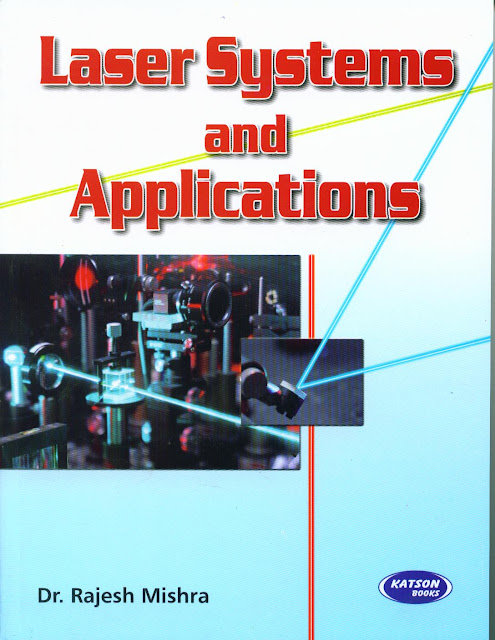• www.uptuexam.com
• GBTU Results 2014
• MTU Results 2014
• UPTU Circulars
• COP Result 2012,13,14
• UPTU Previous Papers
• GATE study material
• Robotics Projects
Thank you for visiting www.UPTUexam.com " Free download Exam materials, previous year question papers, free ebooks & Providing Important Questions To Do their Exams Well and Totally a FREE SERVICE for all engineering streams (B.Tech)."

### Laser Systems and Applications

Laser Systems and Applications :- Important questions1)    Explain the types of coherence
2)    Explain the types of emission
3)    Explain Gain, Gain clamping, Gain efficiency, Absorption.
4)   Explain population inversion.
5)   The coherence length of sodium light is 2.545x10-2m and its wavelength is 5890A0. Calculate
frequency and coherence time.
6)   Discuss the spatial coherence as related to the size of the source. Obtain expression for lateral
width and give its significance.

7)   Deduce the time -independent Schrodinger’s wave equation.

8)   Explain the different types of pumping techniques.

9)   Derive the relation between Einstein’s coefficient.

10)   Explain the characteristics of laser?

11)  What is an optical resonant cavity? What role does it play in a laser?

12)  Discuss de-Broglie theory of matter waves.
13)   Derive an expression for de-Broglie wavelength.
14)  Explain principle, construction and working of Fabry –Parrot resonator.
15)   Discuss on the quantum physics briefly.
16)   Deduce the time -dependent Schrodinger’s wave equation.
17)  What is uncertainty principle? Apply it to prove the non existence of electron in the nucleus.
18)   What is Compton effect? Derive an equation for Compton shift.
19)   Discuss the dual nature of matter and waves.
20)   Calculate the population ratio of two states in laser that produces light of wavelength 6000A0             at3000 K.